ICSE Class 10 Maths Sample Question Paper – Mock 2 with Answers are given at BYJU’S that can be downloaded in a pdf format and also can be accessed online. The best way to crack the ICSE Exam is to practise more sample papers for ICSE class 10 maths, so that it will be easy to analyse the pattern and difficulty level of questions that appear in the exam.

Students can refer to the multiple sample papers at BYJU’S, along with the board papers. This will help them in practising a variety of papers of ICSE to improve their confidence level. They can also get Mock Sample Paper 2 of ICSE Class 10 Maths, along with detailed explanations prepared by our experts.

#### ICSE Class 10 Maths Mock Sample Paper 2 with Solutions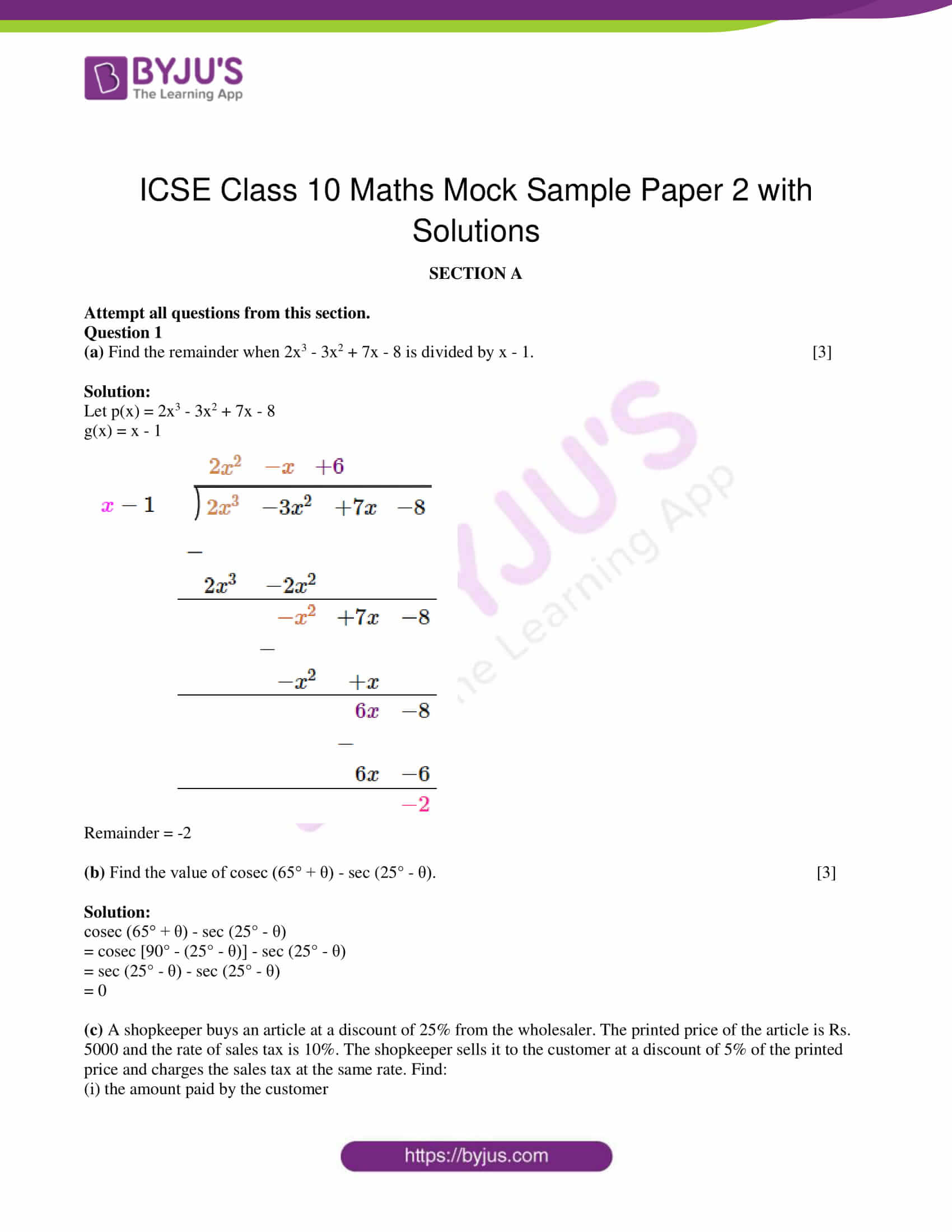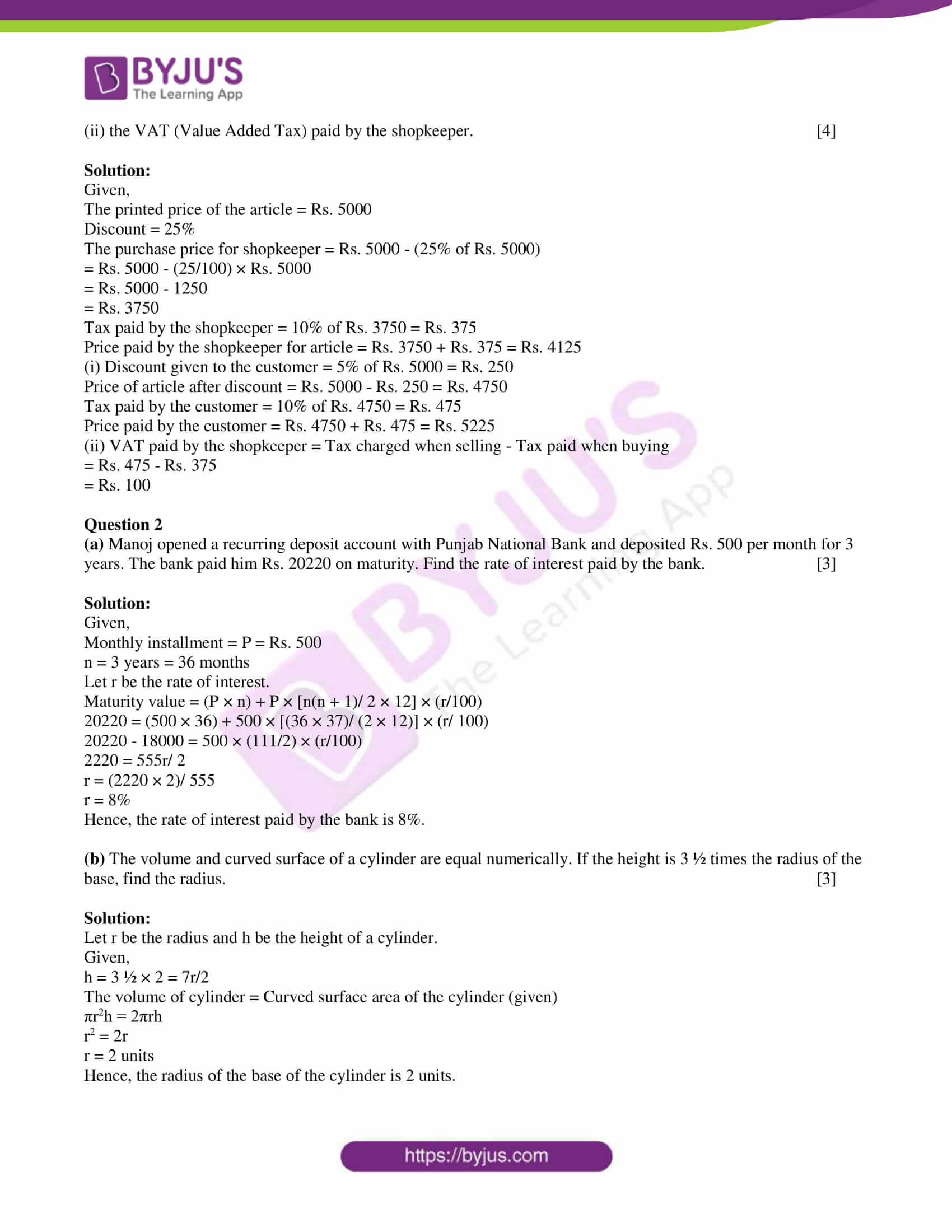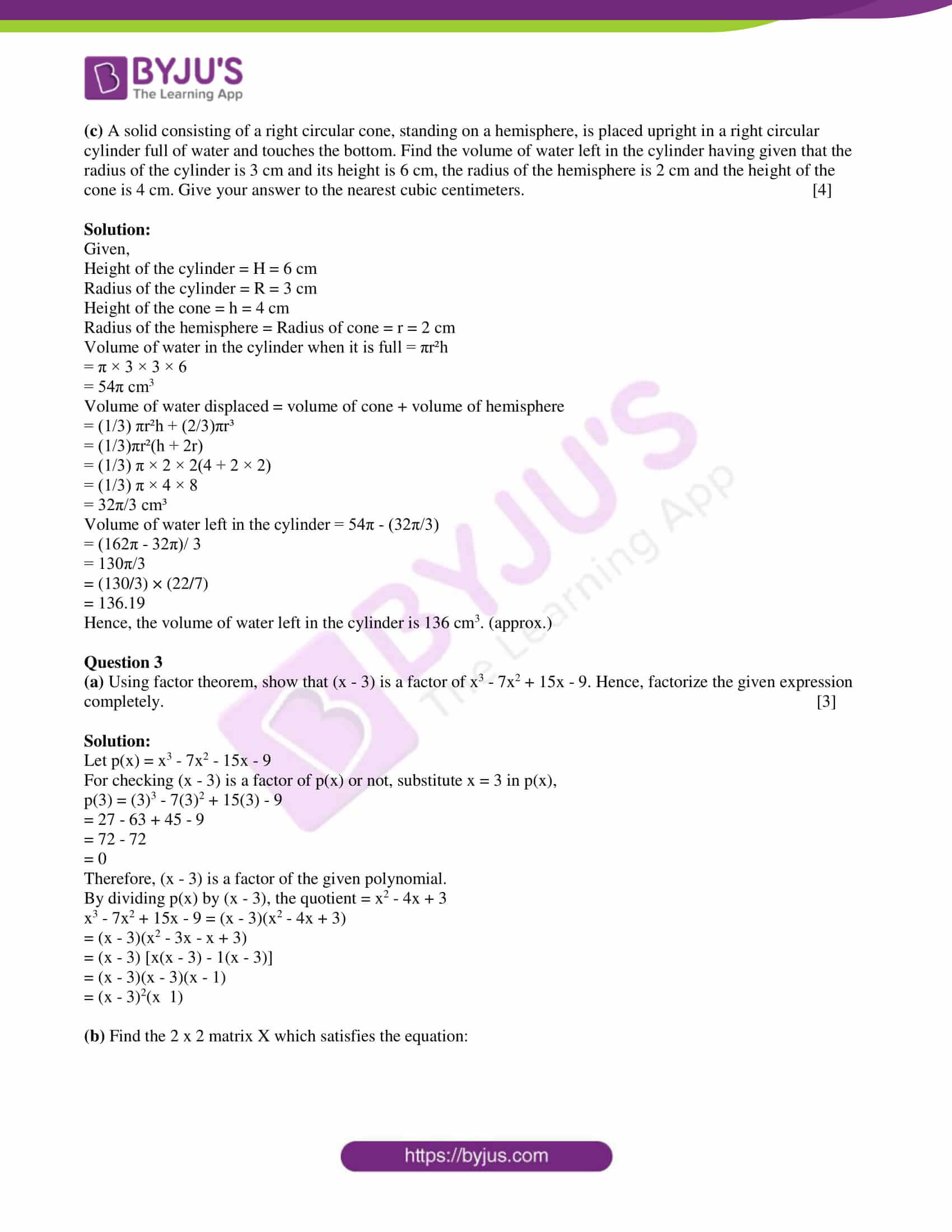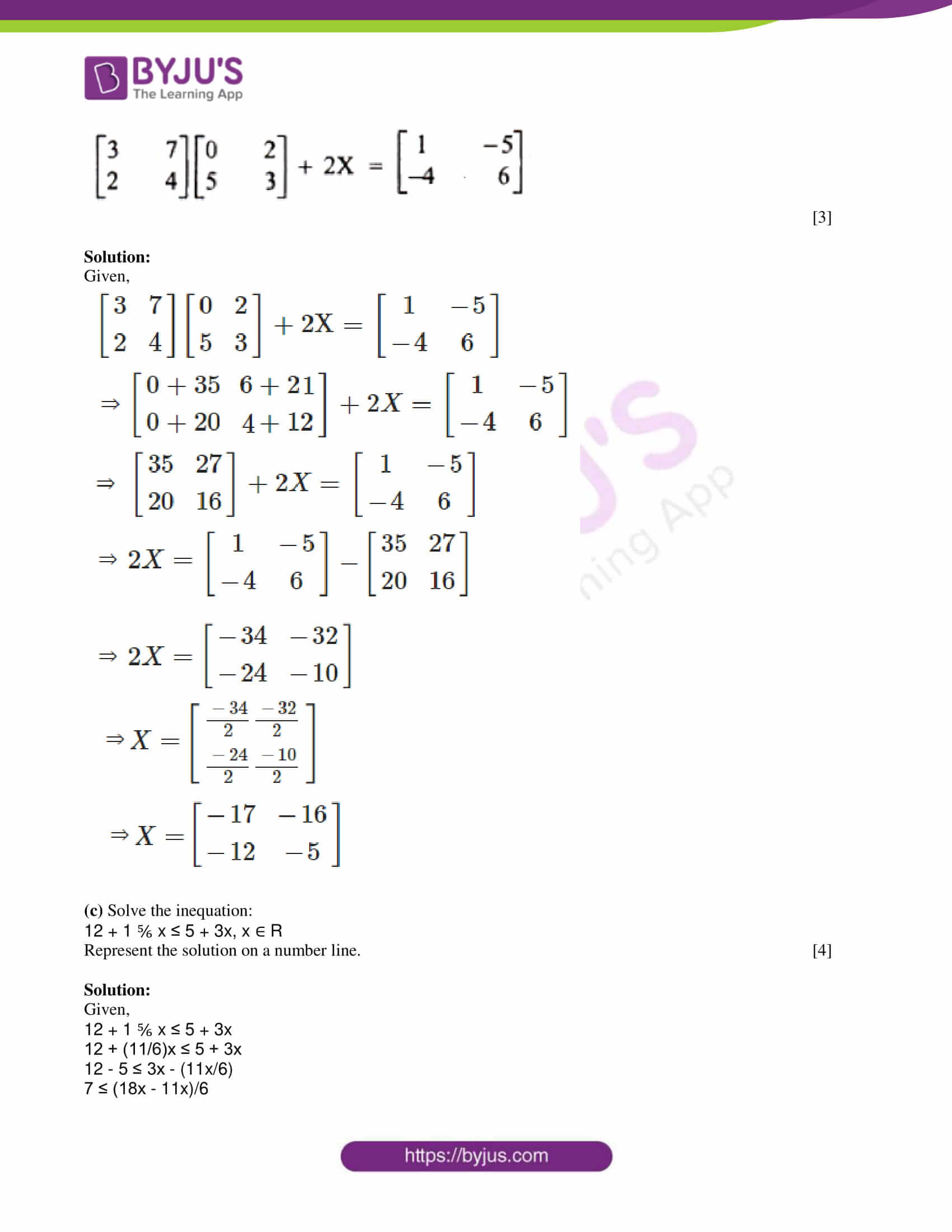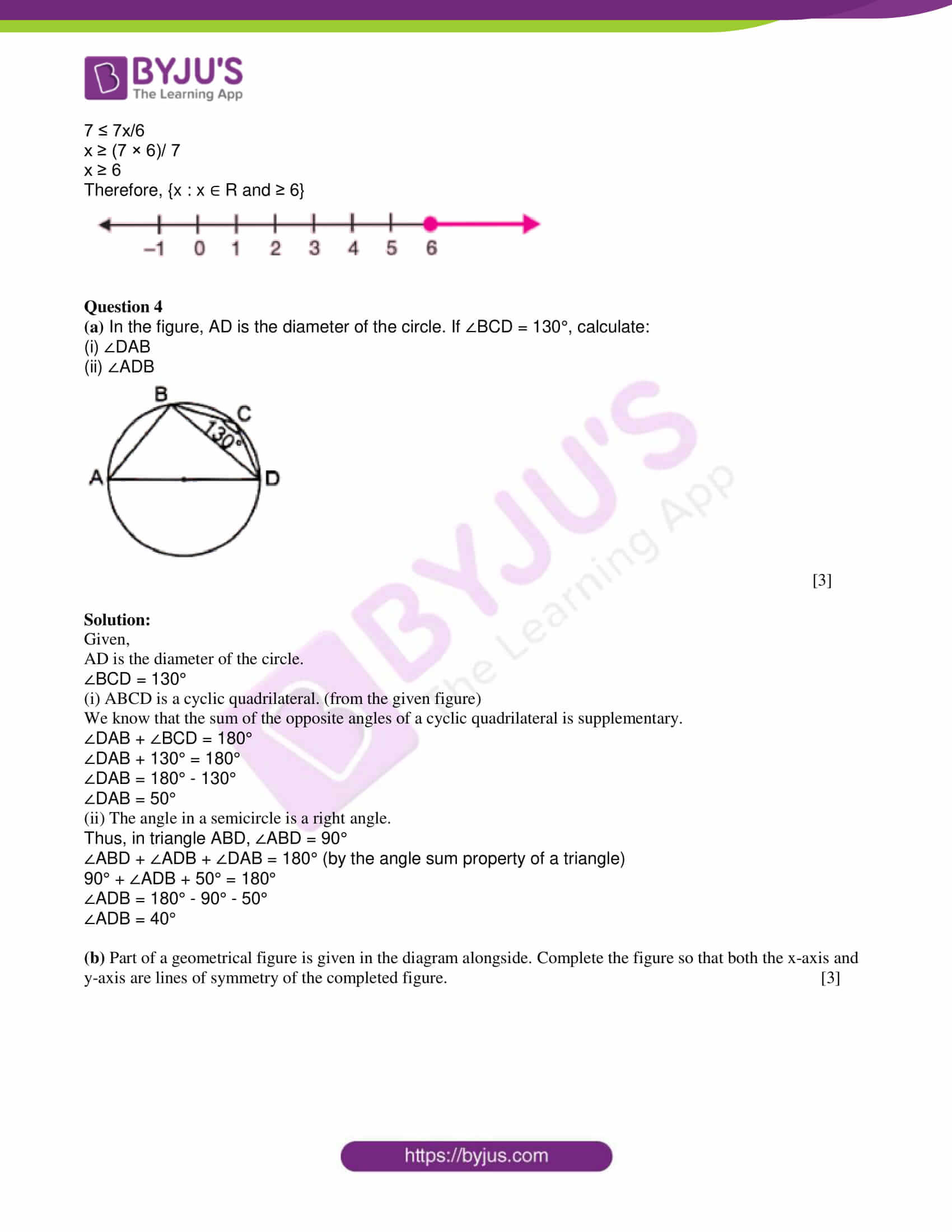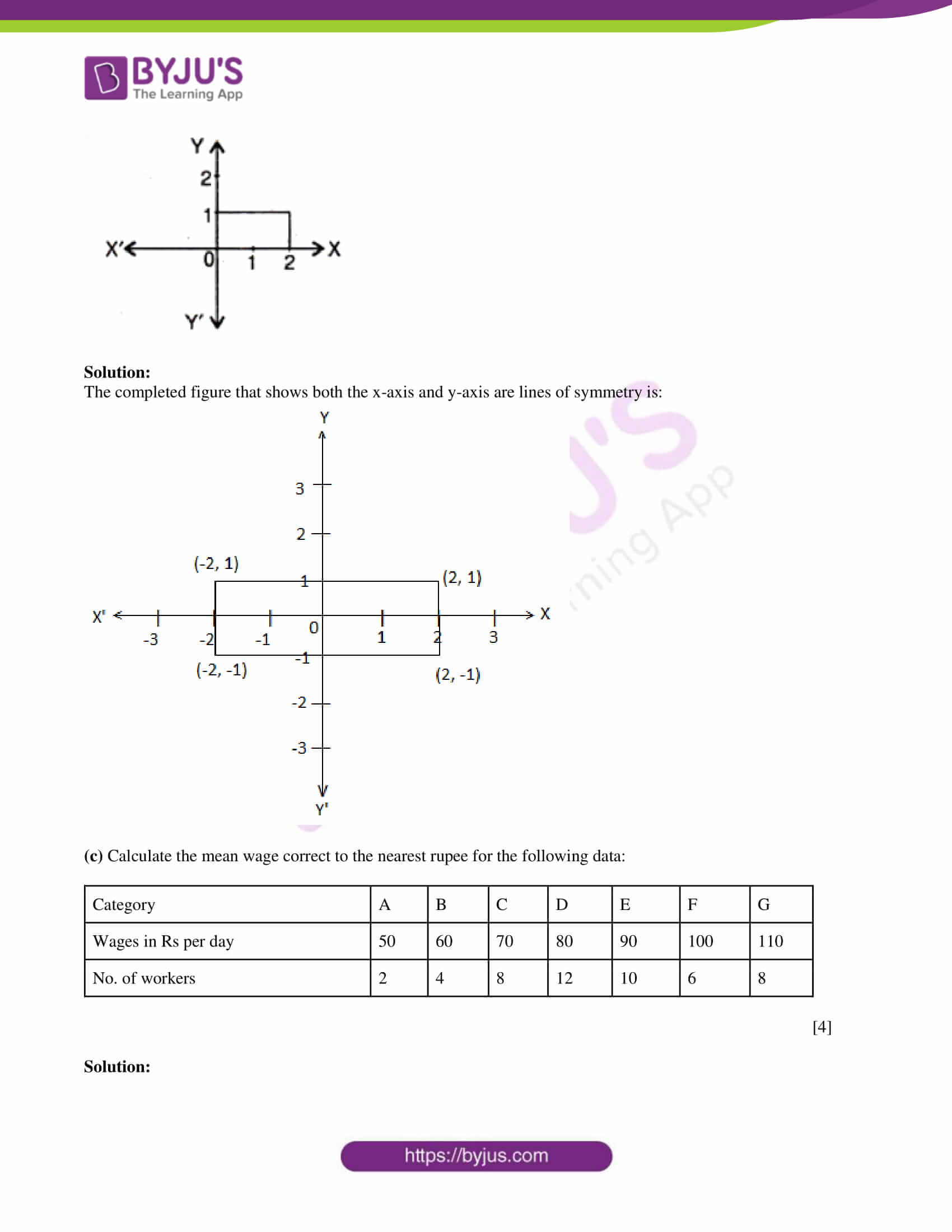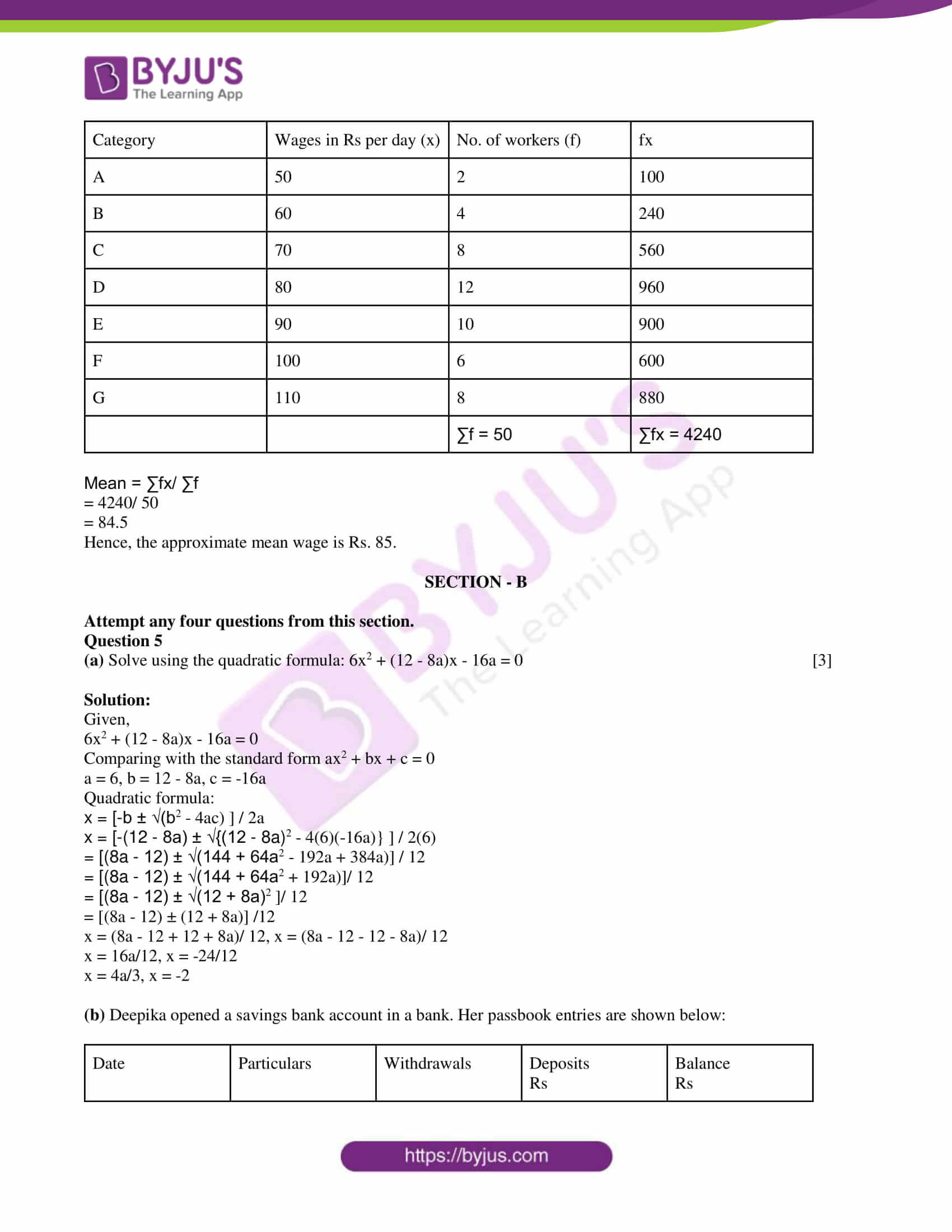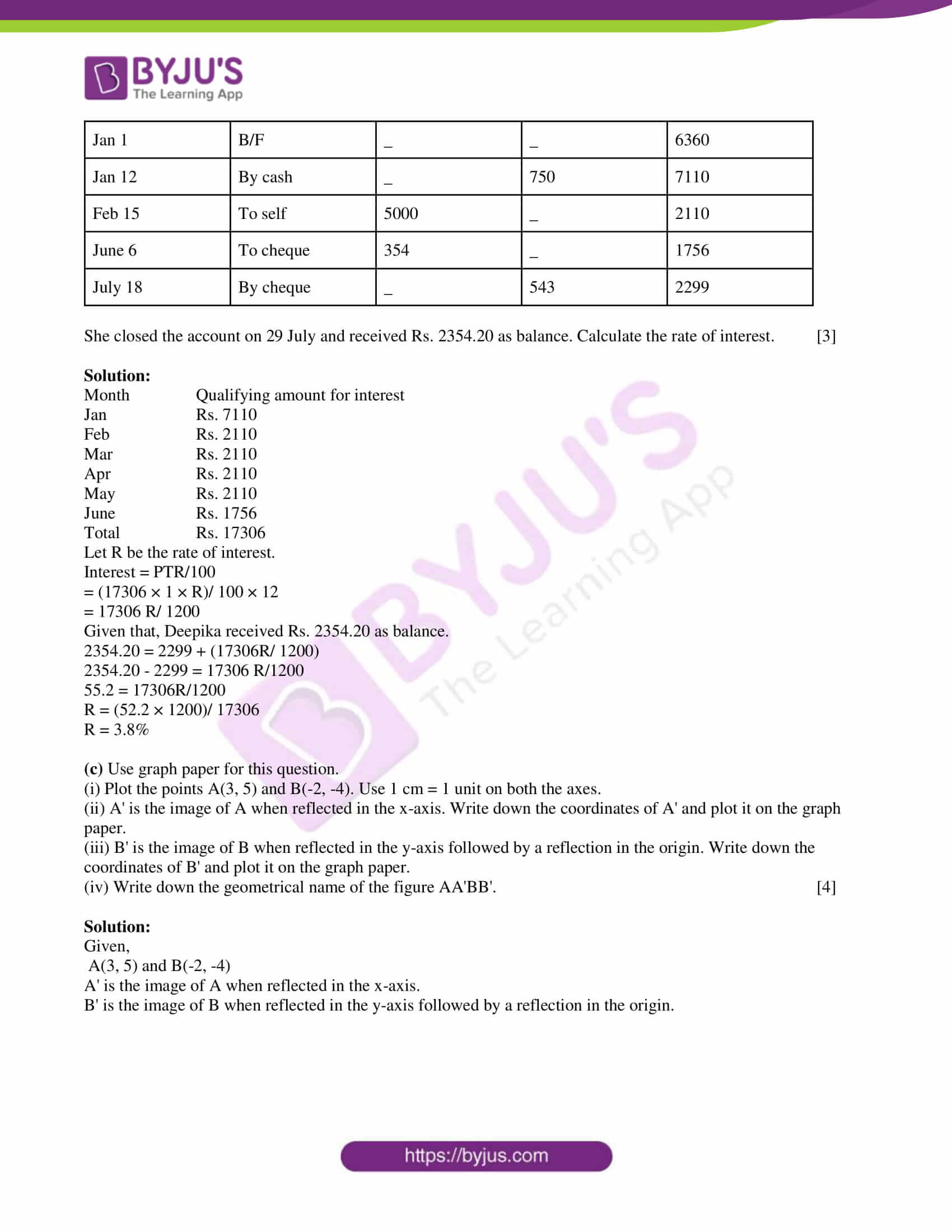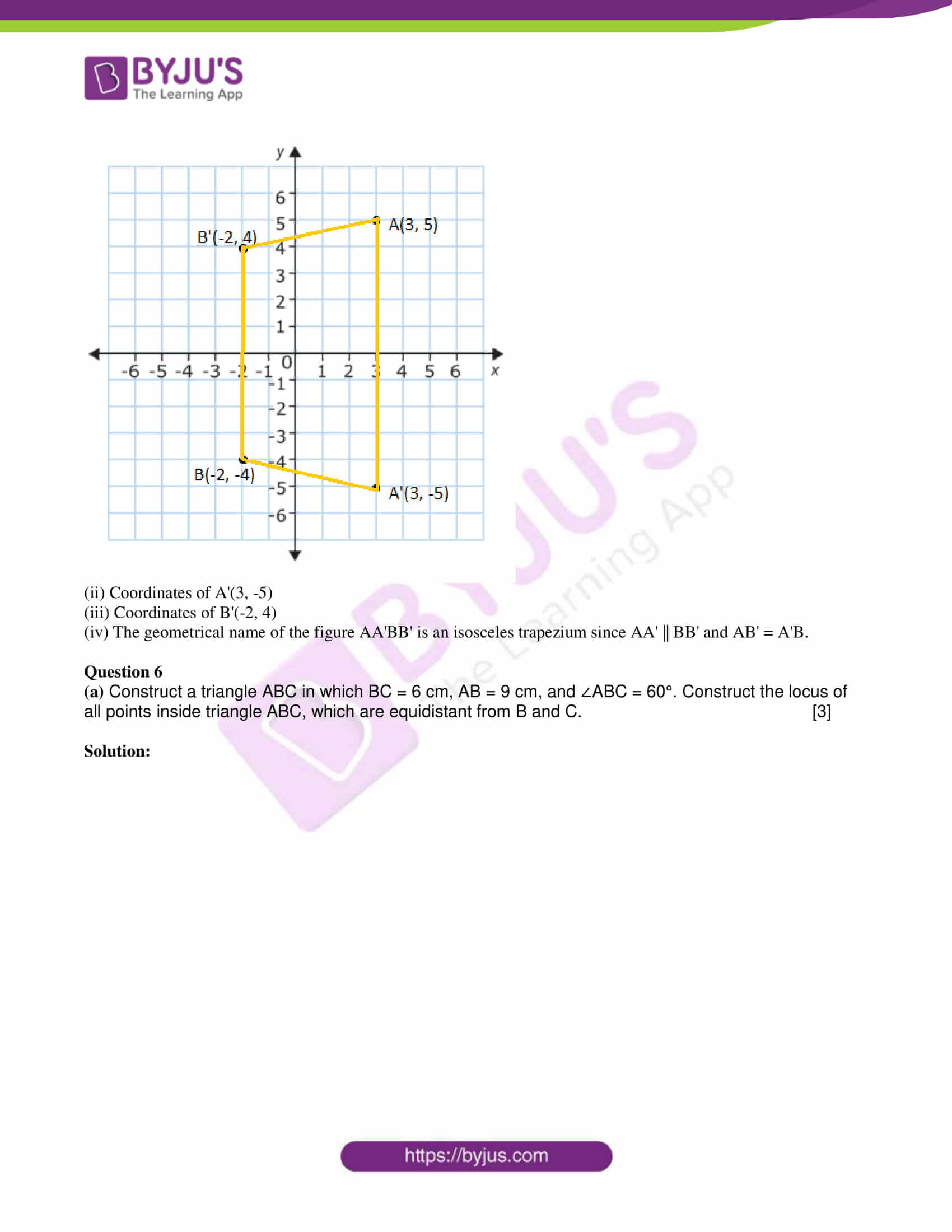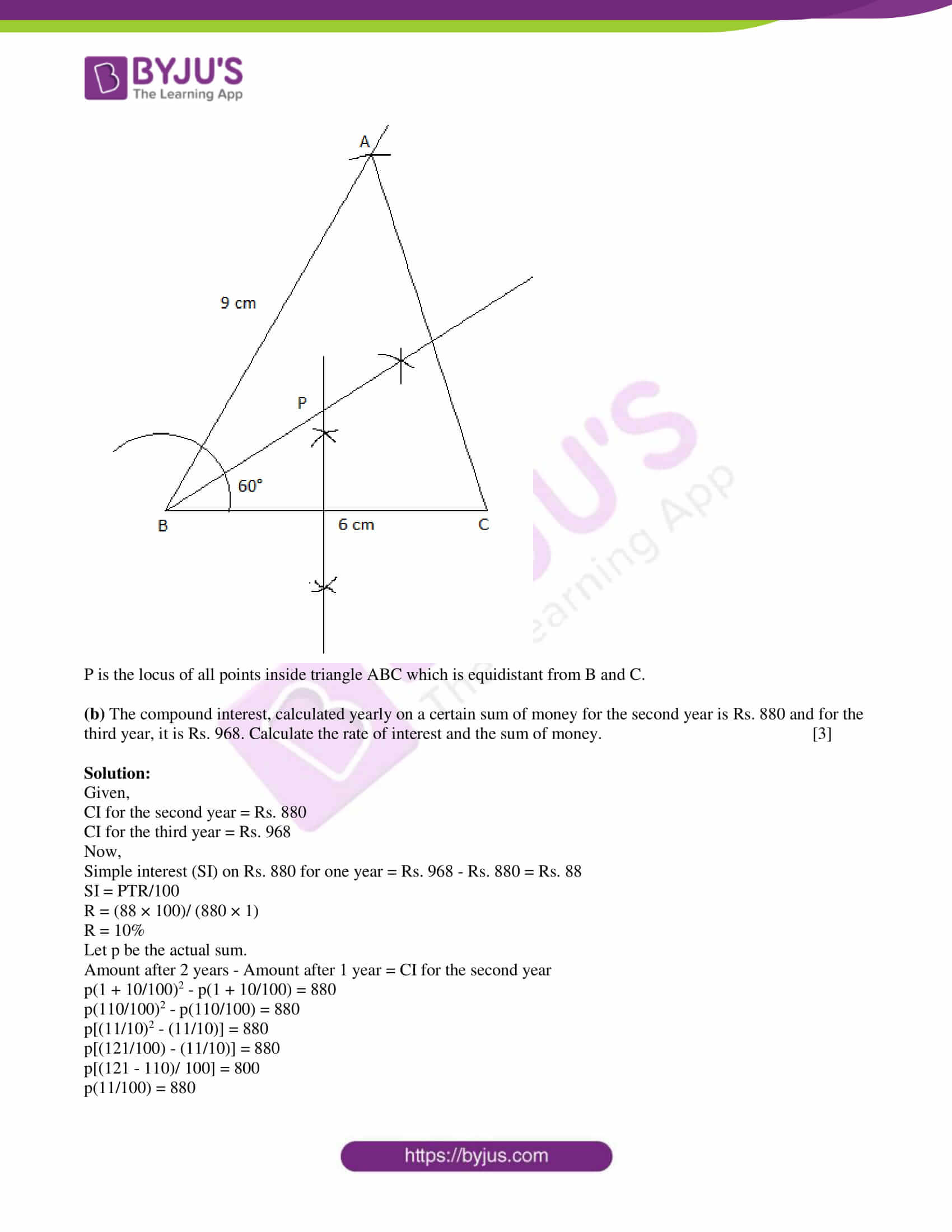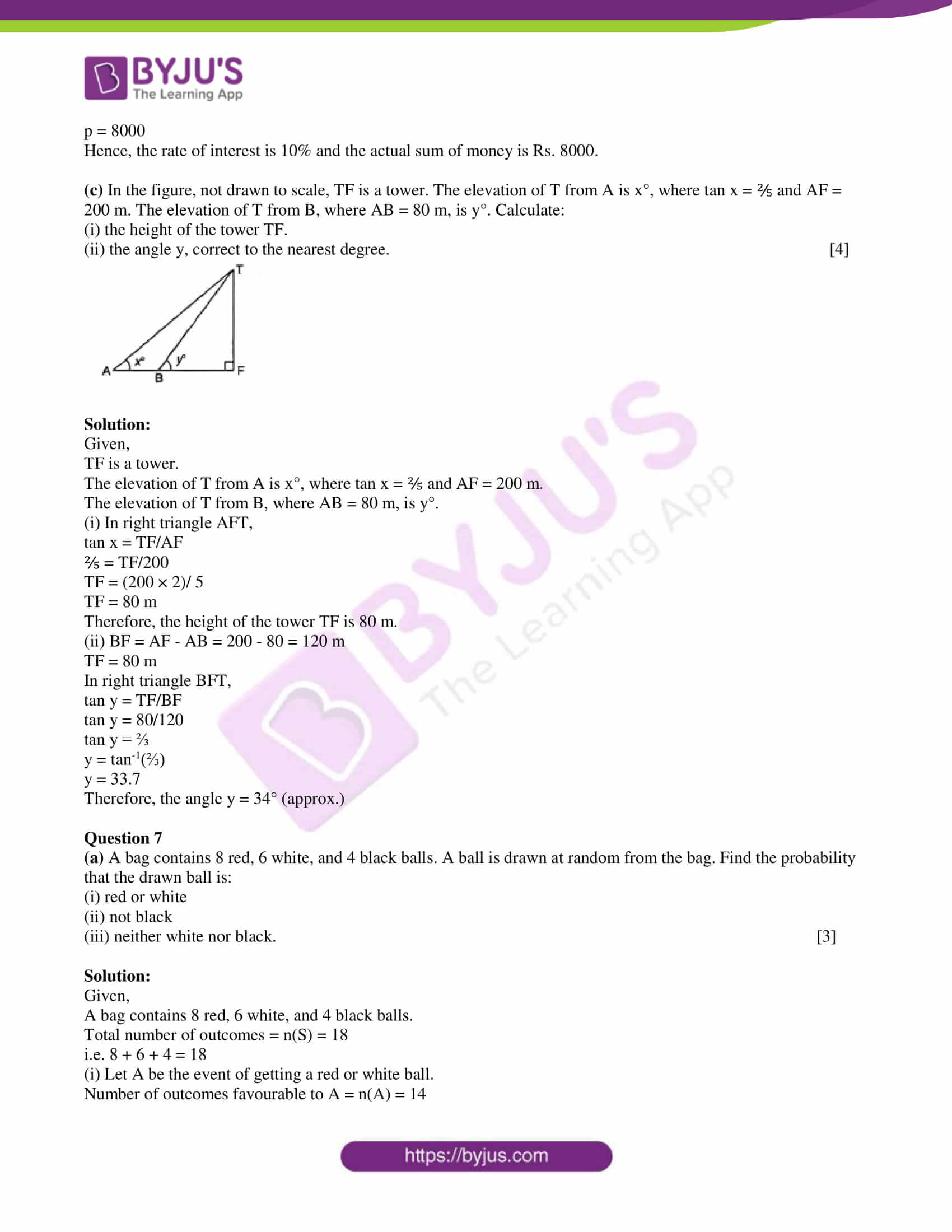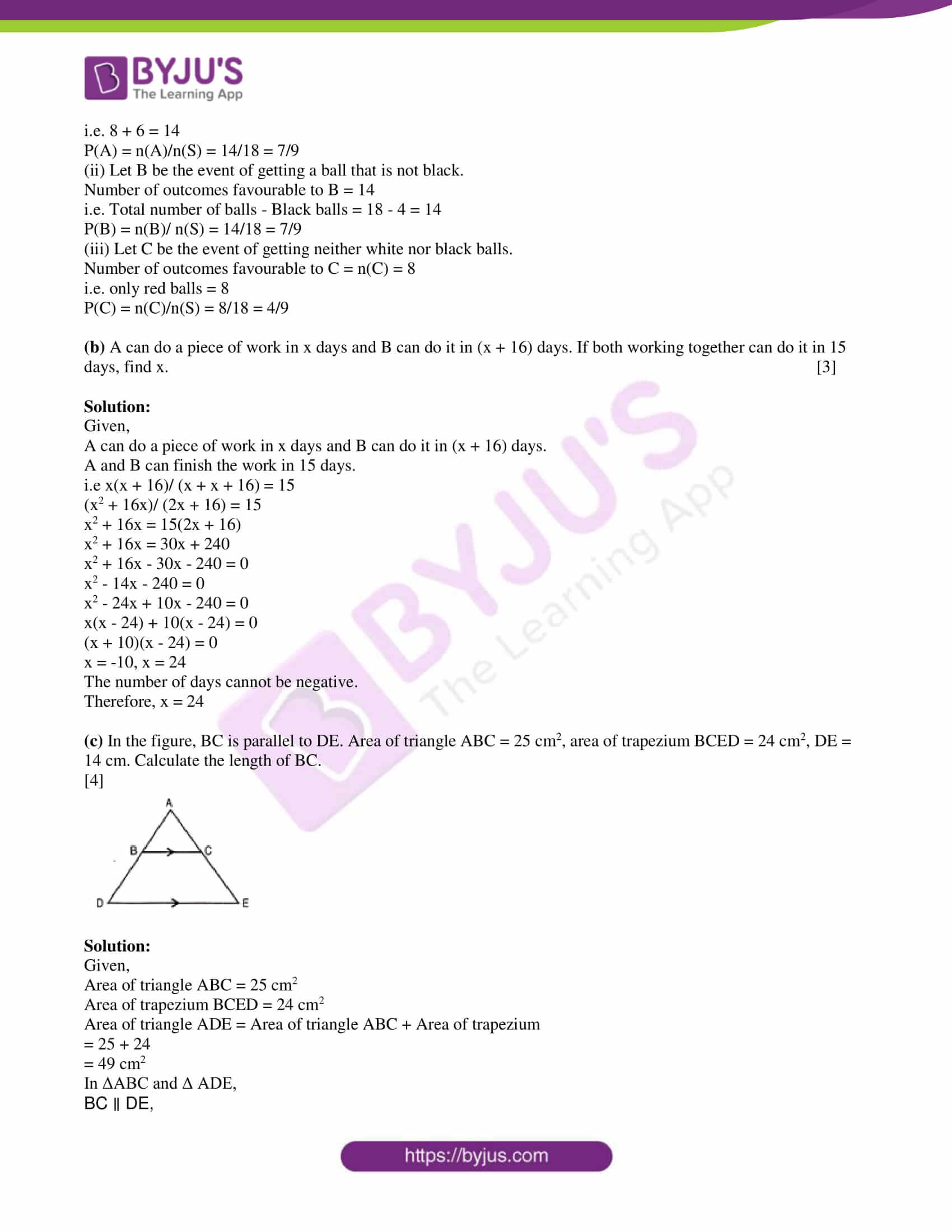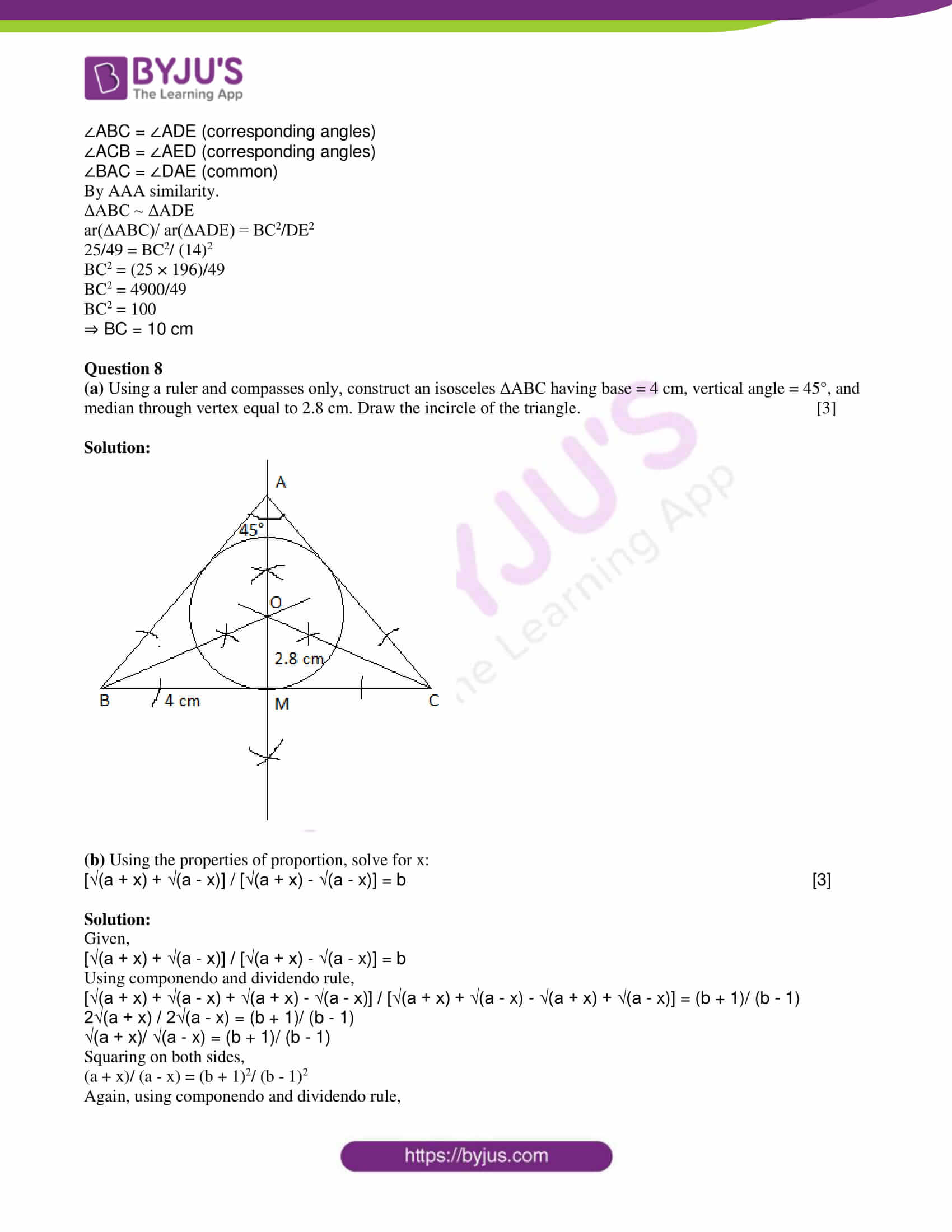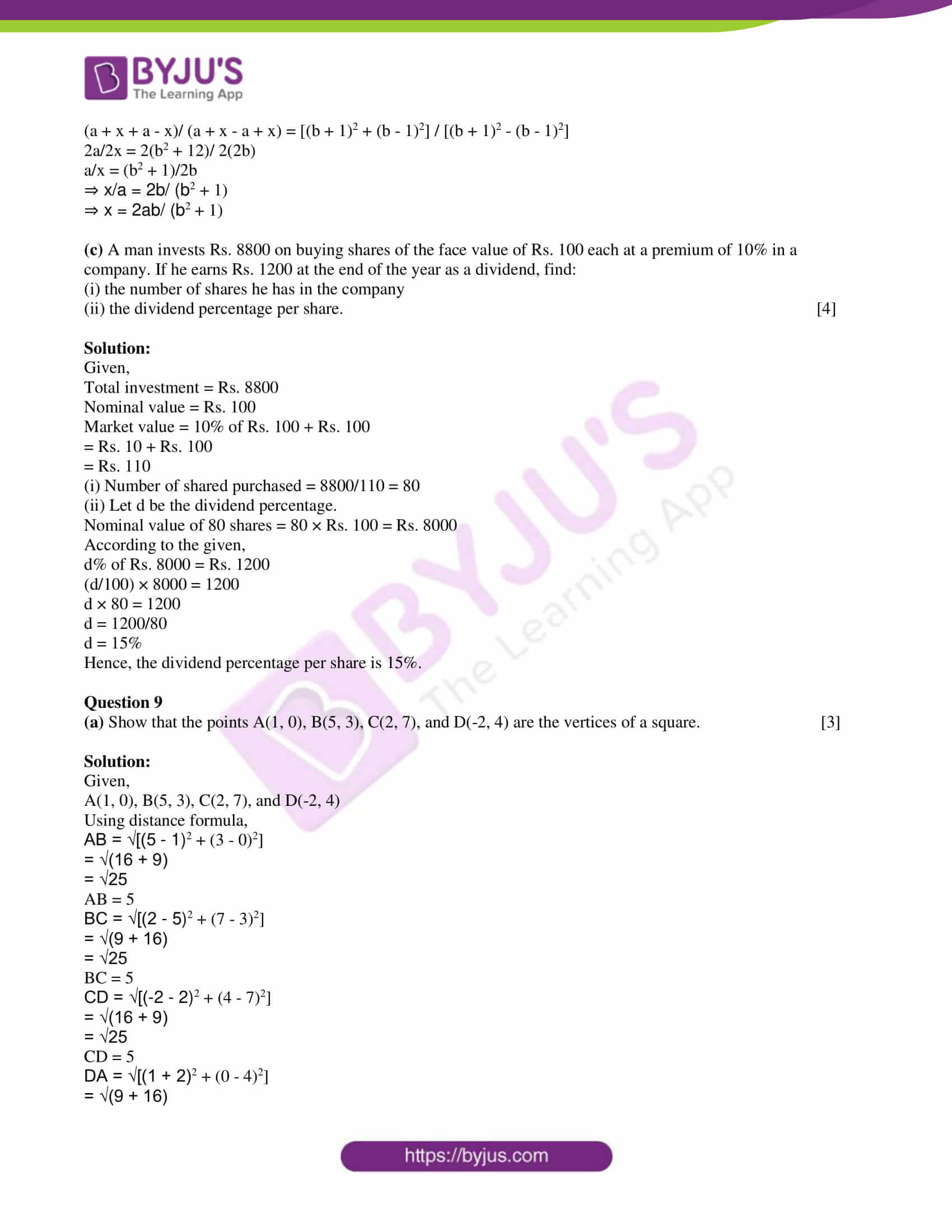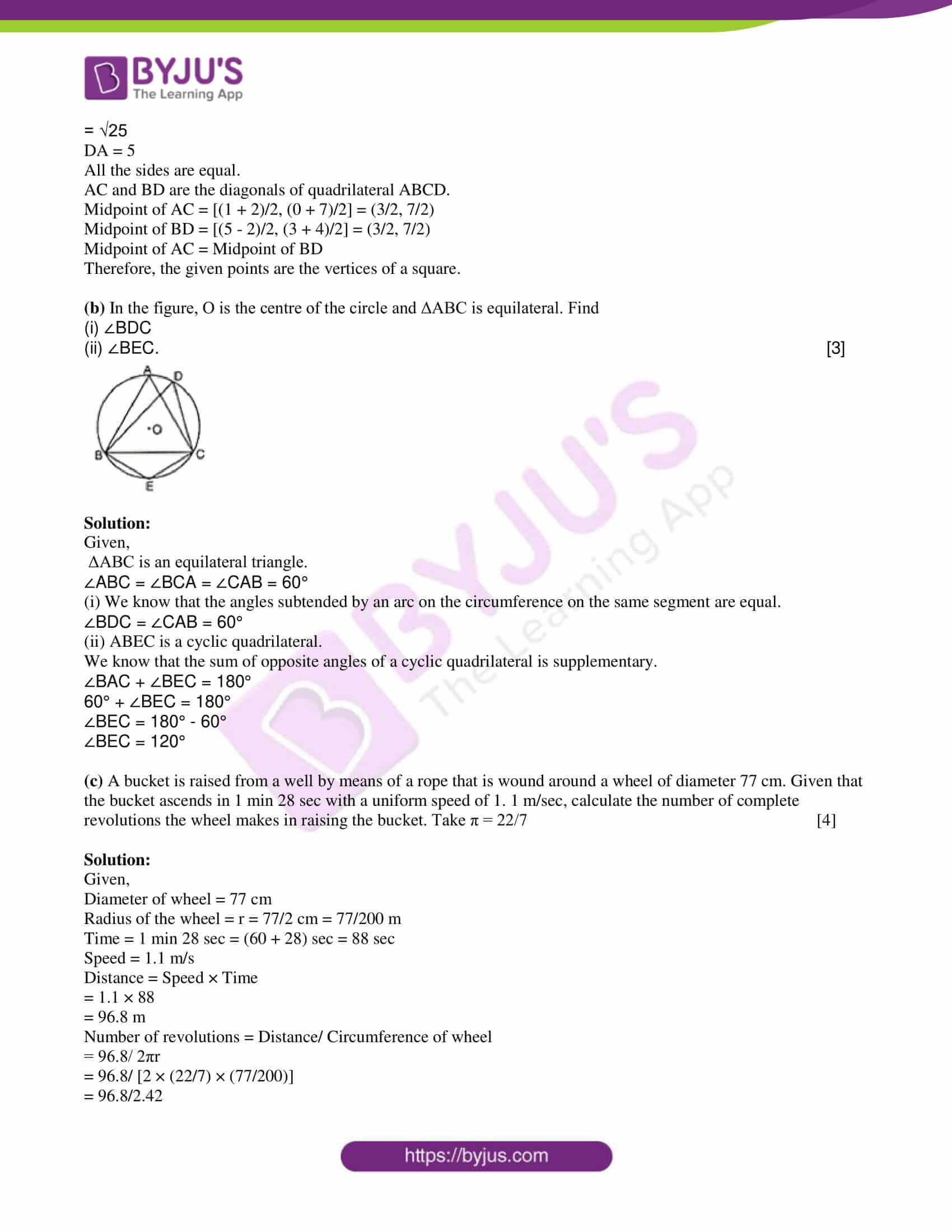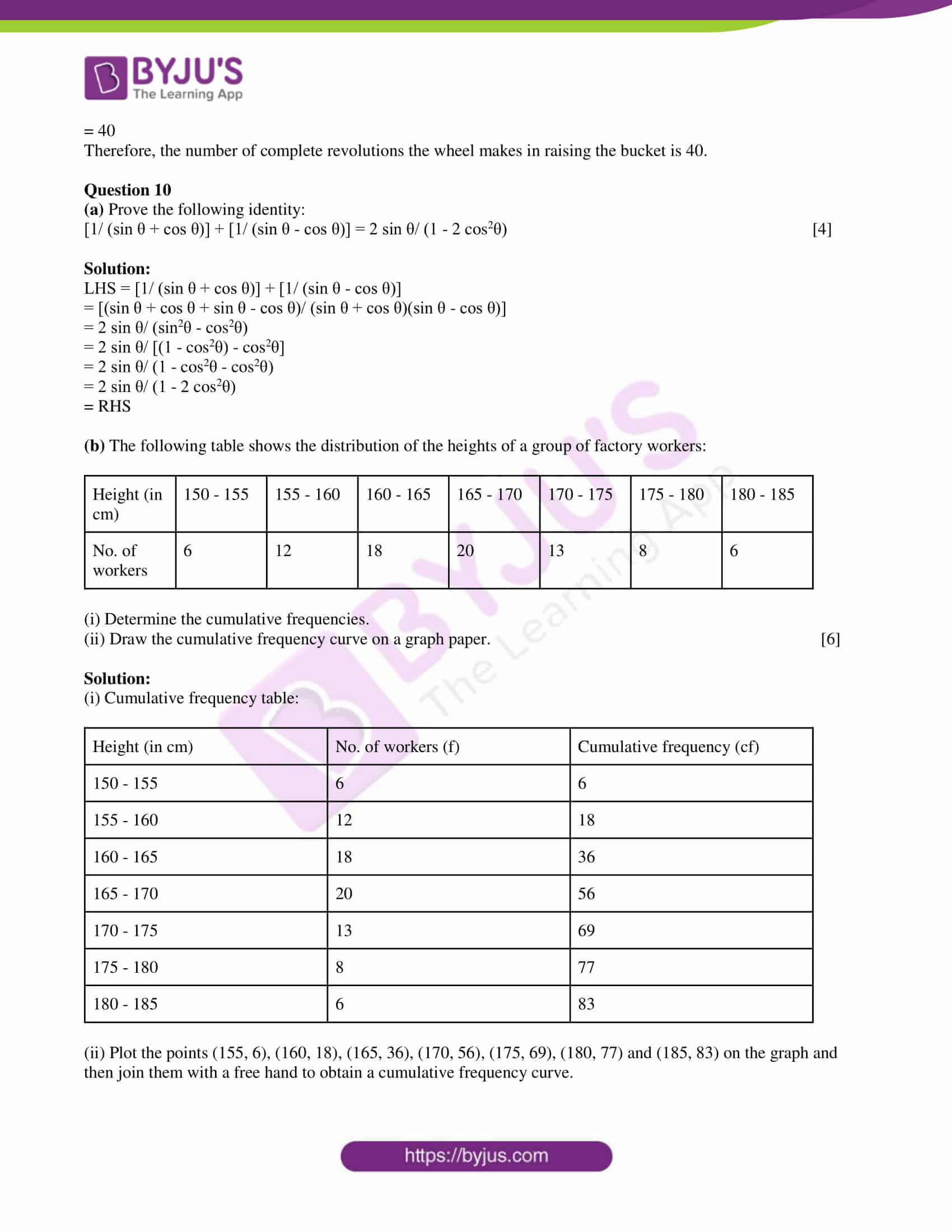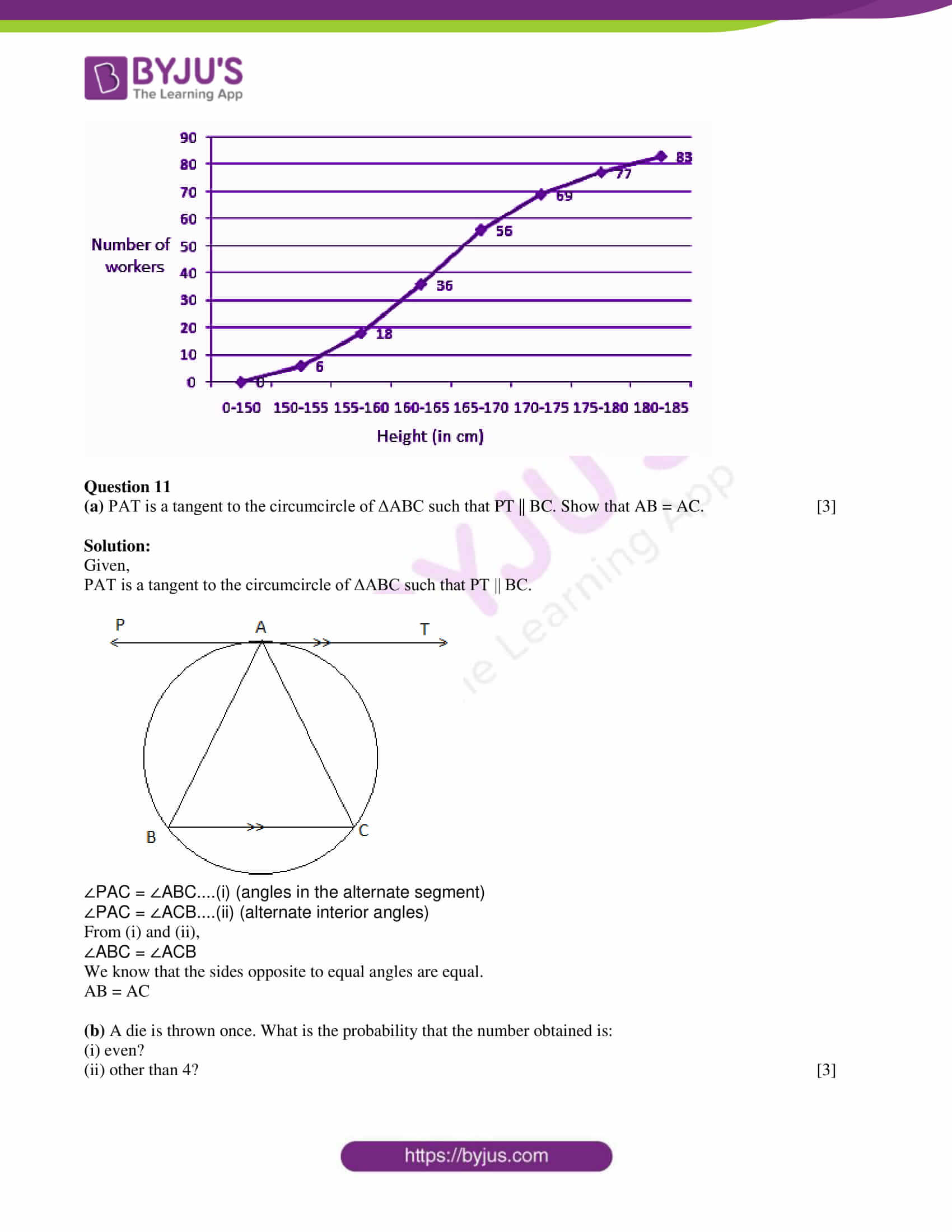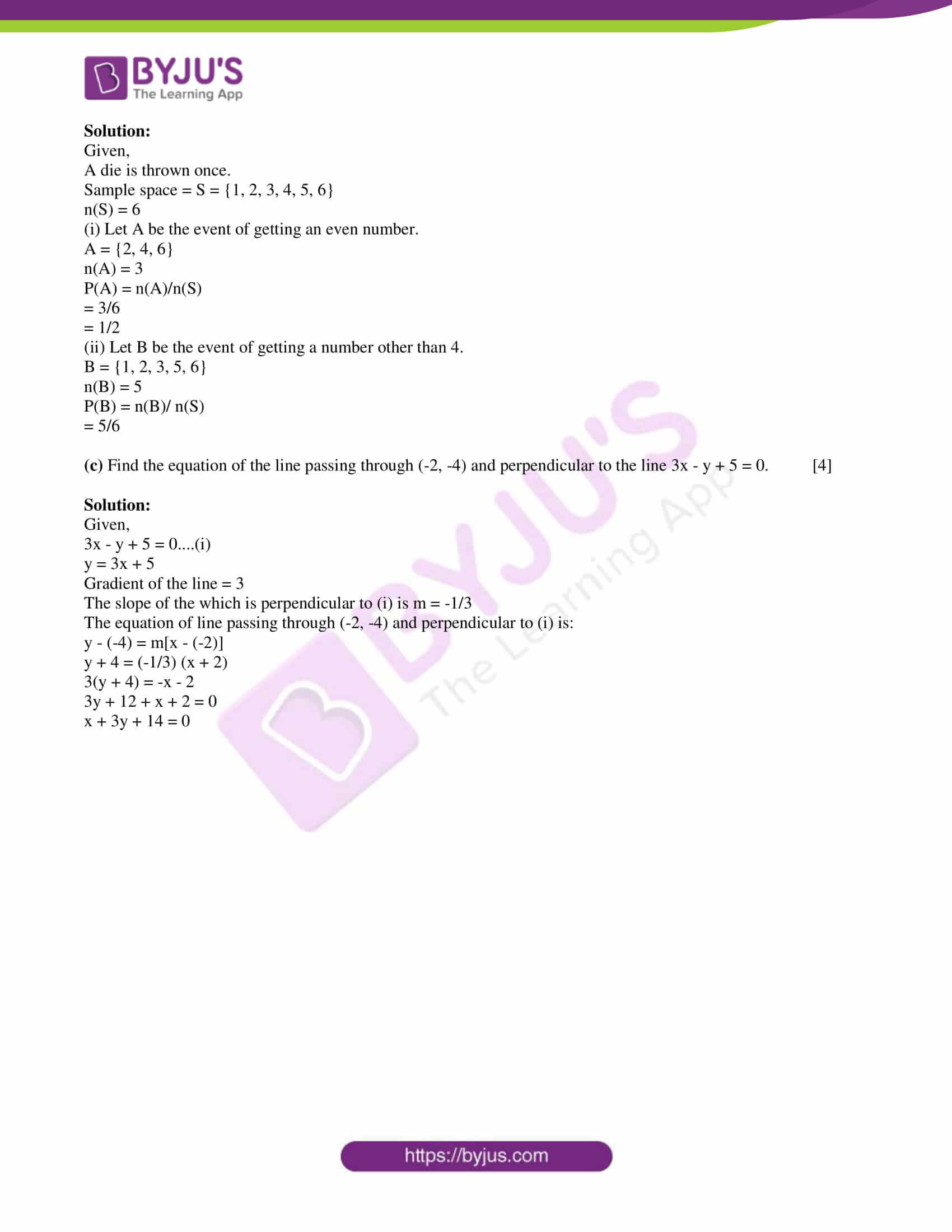SECTION A

Attempt all questions from this section.

Question 1

(a) Find the remainder when 2x3 – 3x2 + 7x – 8 is divided by x – 1. 

Solution:

Let p(x) = 2x3 – 3x2 + 7x – 8

g(x) = x – 1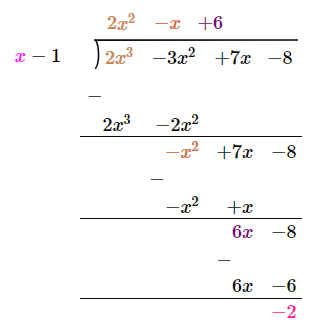Remainder = -2

(b) Find the value of cosec (65° + θ) – sec (25° – θ). 

Solution:

cosec (65° + θ) – sec (25° – θ)

= cosec [90° – (25° – θ)] – sec (25° – θ)

= sec (25° – θ) – sec (25° – θ)

= 0

(c) A shopkeeper buys an article at a discount of 25% from the wholesaler. The printed price of the article is Rs. 5000 and the rate of sales tax is 10%. The shopkeeper sells it to the customer at a discount of 5% of the printed price and charges the sales tax at the same rate. Find:

(i) the amount paid by the customer

(ii) the VAT (Value Added Tax) paid by the shopkeeper. 

Solution:

Given,

The printed price of the article = Rs. 5000

Discount = 25%

The purchase price for shopkeeper = Rs. 5000 – (25% of Rs. 5000)

= Rs. 5000 – (25/100) × Rs. 5000

= Rs. 5000 – 1250

= Rs. 3750

Tax paid by the shopkeeper = 10% of Rs. 3750 = Rs. 375

Price paid by the shopkeeper for article = Rs. 3750 + Rs. 375 = Rs. 4125

(i) Discount given to the customer = 5% of Rs. 5000 = Rs. 250

Price of article after discount = Rs. 5000 – Rs. 250 = Rs. 4750

Tax paid by the customer = 10% of Rs. 4750 = Rs. 475

Price paid by the customer = Rs. 4750 + Rs. 475 = Rs. 5225

(ii) VAT paid by the shopkeeper = Tax charged when selling – Tax paid when buying

= Rs. 475 – Rs. 375

= Rs. 100

Question 2

(a) Manoj opened a recurring deposit account with Punjab National Bank and deposited Rs. 500 per month for 3 years. The bank paid him Rs. 20220 on maturity. Find the rate of interest paid by the bank. 

Solution:

Given,

Monthly installment = P = Rs. 500

n = 3 years = 36 months

Let r be the rate of interest.

Maturity value = (P × n) + P × [n(n + 1)/ 2 × 12] × (r/100)

20220 = (500 × 36) + 500 × [(36 × 37)/ (2 × 12)] × (r/ 100)

20220 – 18000 = 500 × (111/2) × (r/100)

2220 = 555r/ 2

r = (2220 × 2)/ 555

r = 8%

Hence, the rate of interest paid by the bank is 8%.

(b) The volume and curved surface of a cylinder are equal numerically. If the height is 3 ½ times the radius of the base, find the radius. 

Solution:

Let r be the radius and h be the height of a cylinder.

Given,

h = 3 ½ × 2 = 7r/2

The volume of cylinder = Curved surface area of the cylinder (given)

πr2h = 2πrh

r2 = 2r

r = 2 units

Hence, the radius of the base of the cylinder is 2 units.

(c) A solid consisting of a right circular cone, standing on a hemisphere, is placed upright in a right circular cylinder full of water and touches the bottom. Find the volume of water left in the cylinder having given that the radius of the cylinder is 3 cm and its height is 6 cm, the radius of the hemisphere is 2 cm and the height of the cone is 4 cm. Give your answer to the nearest cubic centimeters. 

Solution:

Given,

Height of the cylinder = H = 6 cm

Radius of the cylinder = R = 3 cm

Height of the cone = h = 4 cm

Radius of the hemisphere = Radius of cone = r = 2 cm

Volume of water in the cylinder when it is full = πr²h

= π × 3 × 3 × 6

= 54π cm3

Volume of water displaced = volume of cone + volume of hemisphere

= (1/3) πr²h + (2/3)πr³

= (1/3)πr²(h + 2r)

= (1/3) π × 2 × 2(4 + 2 × 2)

= (1/3) π × 4 × 8

= 32π/3 cm³

Volume of water left in the cylinder = 54π – (32π/3)

= (162π – 32π)/ 3

= 130π/3

= (130/3) × (22/7)

= 136.19

Hence, the volume of water left in the cylinder is 136 cm3. (approx.)

Question 3

(a) Using factor theorem, show that (x – 3) is a factor of x3 – 7x2 + 15x – 9. Hence, factorize the given expression completely. 

Solution:

Let p(x) = x3 – 7x2 – 15x – 9

For checking (x – 3) is a factor of p(x) or not, substitute x = 3 in p(x),

p(3) = (3)3 – 7(3)2 + 15(3) – 9

= 27 – 63 + 45 – 9

= 72 – 72

= 0

Therefore, (x – 3) is a factor of the given polynomial.

By dividing p(x) by (x – 3), the quotient = x2 – 4x + 3

x3 – 7x2 + 15x – 9 = (x – 3)(x2 – 4x + 3)

= (x – 3)(x2 – 3x – x + 3)

= (x – 3) [x(x – 3) – 1(x – 3)]

= (x – 3)(x – 3)(x – 1)

= (x – 3)2(x 1)

(b) Find the 2 x 2 matrix X which satisfies the equation: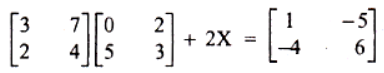Solution:

Given,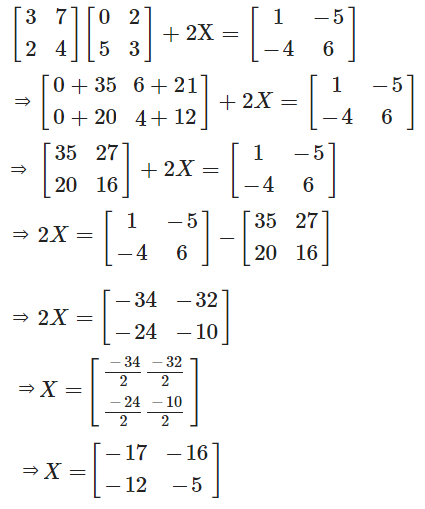(c) Solve the inequation:

12 + 1 ⅚ x ≤ 5 + 3x, x ∈ R

Represent the solution on a number line. 

Solution:

Given,

12 + 1 ⅚ x ≤ 5 + 3x

12 + (11/6)x ≤ 5 + 3x

12 – 5 ≤ 3x – (11x/6)

7 ≤ (18x – 11x)/6

7 ≤ 7x/6

x ≥ (7 × 6)/ 7

x ≥ 6

Therefore, {x : x ∈ R and ≥ 6}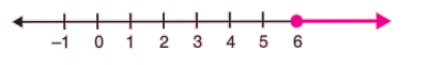Question 4

(a) In the figure, AD is the diameter of the circle. If ∠BCD = 130°, calculate:

(i) ∠DAB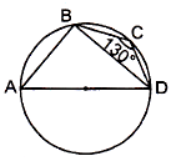Solution:

Given,

AD is the diameter of the circle.

∠BCD = 130°

(i) ABCD is a cyclic quadrilateral. (from the given figure)

We know that the sum of the opposite angles of a cyclic quadrilateral is supplementary.

∠DAB + ∠BCD = 180°

∠DAB + 130° = 180°

∠DAB = 180° – 130°

∠DAB = 50°

(ii) The angle in a semicircle is a right angle.

Thus, in triangle ABD, ∠ABD = 90°

∠ABD + ∠ADB + ∠DAB = 180° (by the angle sum property of a triangle)

90° + ∠ADB + 50° = 180°

∠ADB = 180° – 90° – 50°

(b) Part of a geometrical figure is given in the diagram alongside. Complete the figure so that both the x-axis and y-axis are lines of symmetry of the completed figure.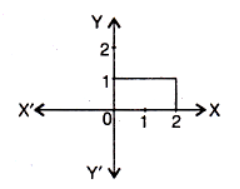Solution:

The completed figure that shows both the x-axis and y-axis are lines of symmetry is: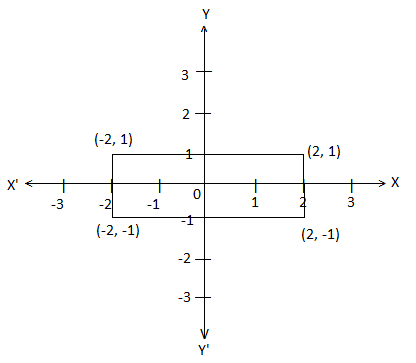(c) Calculate the mean wage correct to the nearest rupee for the following data:

 Category A B C D E F G Wages in Rs per day 50 60 70 80 90 100 110 No. of workers 2 4 8 12 10 6 8


Solution:

 Category Wages in Rs per day (x) No. of workers (f) fx A 50 2 100 B 60 4 240 C 70 8 560 D 80 12 960 E 90 10 900 F 100 6 600 G 110 8 880 ∑f = 50 ∑fx = 4240

Mean = ∑fx/ ∑f

= 4240/ 50

= 84.5

Hence, the approximate mean wage is Rs. 85.

SECTION – B

Attempt any four questions from this section.

Question 5

(a) Solve using the quadratic formula: 6x2 + (12 – 8a)x – 16a = 0 

Solution:

Given,

6x2 + (12 – 8a)x – 16a = 0

Comparing with the standard form ax2 + bx + c = 0

a = 6, b = 12 – 8a, c = -16a

x = [-b ± √(b2 – 4ac) ] / 2a

x = [-(12 – 8a) ± √{(12 – 8a)2 – 4(6)(-16a)} ] / 2(6)

= [(8a – 12) ± √(144 + 64a2 – 192a + 384a)] / 12

= [(8a – 12) ± √(144 + 64a2 + 192a)]/ 12

= [(8a – 12) ± √(12 + 8a)2 ]/ 12

= [(8a – 12) ± (12 + 8a)] /12

x = (8a – 12 + 12 + 8a)/ 12, x = (8a – 12 – 12 – 8a)/ 12

x = 16a/12, x = -24/12

x = 4a/3, x = -2

(b) Deepika opened a savings bank account in a bank. Her passbook entries are shown below:

 Date Particulars Withdrawals Deposits Rs Balance Rs Jan 1 B/F _ _ 6360 Jan 12 By cash _ 750 7110 Feb 15 To self 5000 _ 2110 June 6 To cheque 354 _ 1756 July 18 By cheque _ 543 2299

She closed the account on 29 July and received Rs. 2354.20 as balance. Calculate the rate of interest. 

Solution:

Month Qualifying amount for interest

Jan Rs. 7110

Feb Rs. 2110

Mar Rs. 2110

Apr Rs. 2110

May Rs. 2110

June Rs. 1756

Total Rs. 17306

Let R be the rate of interest.

Interest = PTR/100

= (17306 × 1 × R)/ 100 × 12

= 17306 R/ 1200

Given that, Deepika received Rs. 2354.20 as balance.

2354.20 = 2299 + (17306R/ 1200)

2354.20 – 2299 = 17306 R/1200

55.2 = 17306R/1200

R = (52.2 × 1200)/ 17306

R = 3.8%

(c) Use graph paper for this question.

(i) Plot the points A(3, 5) and B(-2, -4). Use 1 cm = 1 unit on both the axes.

(ii) A’ is the image of A when reflected in the x-axis. Write down the coordinates of A’ and plot it on the graph paper.

(iii) B’ is the image of B when reflected in the y-axis followed by a reflection in the origin. Write down the coordinates of B’ and plot it on the graph paper.

(iv) Write down the geometrical name of the figure AA’BB’. 

Solution:

Given,

A(3, 5) and B(-2, -4)

A’ is the image of A when reflected in the x-axis.

B’ is the image of B when reflected in the y-axis followed by a reflection in the origin.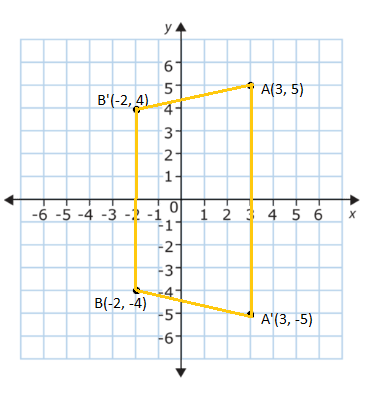(ii) Coordinates of A'(3, -5)

(iii) Coordinates of B'(-2, 4)

(iv) The geometrical name of the figure AA’BB’ is an isosceles trapezium since AA’ || BB’ and AB’ = A’B.

Question 6

(a) Construct a triangle ABC in which BC = 6 cm, AB = 9 cm, and ∠ABC = 60°. Construct the locus of all points inside triangle ABC, which are equidistant from B and C. 

Solution: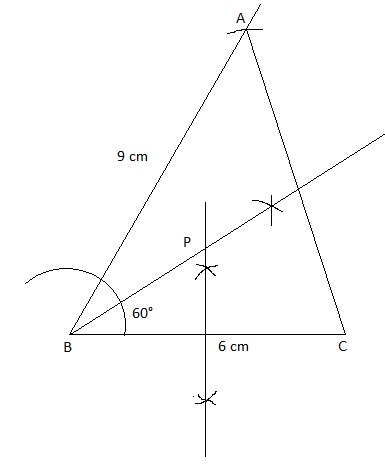P is the locus of all points inside triangle ABC which is equidistant from B and C.

(b) The compound interest, calculated yearly on a certain sum of money for the second year is Rs. 880 and for the third year, it is Rs. 968. Calculate the rate of interest and the sum of money. 

Solution:

Given,

CI for the second year = Rs. 880

CI for the third year = Rs. 968

Now,

Simple interest (SI) on Rs. 880 for one year = Rs. 968 – Rs. 880 = Rs. 88

SI = PTR/100

R = (88 × 100)/ (880 × 1)

R = 10%

Let p be the actual sum.

Amount after 2 years – Amount after 1 year = CI for the second year

p(1 + 10/100)2 – p(1 + 10/100) = 880

p(110/100)2 – p(110/100) = 880

p[(11/10)2 – (11/10)] = 880

p[(121/100) – (11/10)] = 880

p[(121 – 110)/ 100] = 800

p(11/100) = 880

p = 8000

Hence, the rate of interest is 10% and the actual sum of money is Rs. 8000.

(c) In the figure, not drawn to scale, TF is a tower. The elevation of T from A is x°, where tan x = ⅖ and AF = 200 m. The elevation of T from B, where AB = 80 m, is y°. Calculate:

(i) the height of the tower TF.

(ii) the angle y, correct to the nearest degree.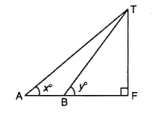Solution:

Given,

TF is a tower.

The elevation of T from A is x°, where tan x = ⅖ and AF = 200 m.

The elevation of T from B, where AB = 80 m, is y°.

(i) In right triangle AFT,

tan x = TF/AF

⅖ = TF/200

TF = (200 × 2)/ 5

TF = 80 m

Therefore, the height of the tower TF is 80 m.

(ii) BF = AF – AB = 200 – 80 = 120 m

TF = 80 m

In right triangle BFT,

tan y = TF/BF

tan y = 80/120

tan y = ⅔

y = tan-1(⅔)

y = 33.7

Therefore, the angle y = 34° (approx.)

Question 7

(a) A bag contains 8 red, 6 white, and 4 black balls. A ball is drawn at random from the bag. Find the probability that the drawn ball is:

(i) red or white

(ii) not black

(iii) neither white nor black. 

Solution:

Given,

A bag contains 8 red, 6 white, and 4 black balls.

Total number of outcomes = n(S) = 18

i.e. 8 + 6 + 4 = 18

(i) Let A be the event of getting a red or white ball.

Number of outcomes favourable to A = n(A) = 14

i.e. 8 + 6 = 14

P(A) = n(A)/n(S) = 14/18 = 7/9

(ii) Let B be the event of getting a ball that is not black.

Number of outcomes favourable to B = 14

i.e. Total number of balls – Black balls = 18 – 4 = 14

P(B) = n(B)/ n(S) = 14/18 = 7/9

(iii) Let C be the event of getting neither white nor black balls.

Number of outcomes favourable to C = n(C) = 8

i.e. only red balls = 8

P(C) = n(C)/n(S) = 8/18 = 4/9

(b) A can do a piece of work in x days and B can do it in (x + 16) days. If both working together can do it in 15 days, find x. 

Solution:

Given,

A can do a piece of work in x days and B can do it in (x + 16) days.

A and B can finish the work in 15 days.

i.e x(x + 16)/ (x + x + 16) = 15

(x2 + 16x)/ (2x + 16) = 15

x2 + 16x = 15(2x + 16)

x2 + 16x = 30x + 240

x2 + 16x – 30x – 240 = 0

x2 – 14x – 240 = 0

x2 – 24x + 10x – 240 = 0

x(x – 24) + 10(x – 24) = 0

(x + 10)(x – 24) = 0

x = -10, x = 24

The number of days cannot be negative.

Therefore, x = 24

(c) In the figure, BC is parallel to DE. Area of triangle ABC = 25 cm2, area of trapezium BCED = 24 cm2, DE = 14 cm. Calculate the length of BC.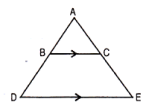Solution:

Given,

Area of triangle ABC = 25 cm2

Area of trapezium BCED = 24 cm2

Area of triangle ADE = Area of triangle ABC + Area of trapezium

= 25 + 24

= 49 cm2

BC ∥ DE,

∠ACB = ∠AED (corresponding angles)

∠BAC = ∠DAE (common)

By AAA similarity.

25/49 = BC2/ (14)2

BC2 = (25 × 196)/49

BC2 = 4900/49

BC2 = 100

⇒ BC = 10 cm

Question 8

(a) Using a ruler and compasses only, construct an isosceles ΔABC having base = 4 cm, vertical angle = 45°, and median through vertex equal to 2.8 cm. Draw the incircle of the triangle. 

Solution: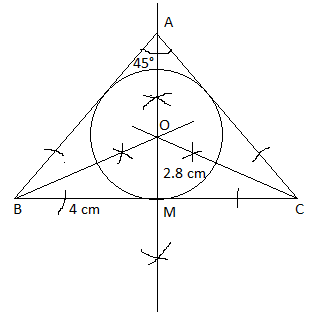(b) Using the properties of proportion, solve for x:

[√(a + x) + √(a – x)] / [√(a + x) – √(a – x)] = b 

Solution:

Given,

[√(a + x) + √(a – x)] / [√(a + x) – √(a – x)] = b

Using componendo and dividendo rule,

[√(a + x) + √(a – x) + √(a + x) – √(a – x)] / [√(a + x) + √(a – x) – √(a + x) + √(a – x)] = (b + 1)/ (b – 1)

2√(a + x) / 2√(a – x) = (b + 1)/ (b – 1)

√(a + x)/ √(a – x) = (b + 1)/ (b – 1)

Squaring on both sides,

(a + x)/ (a – x) = (b + 1)2/ (b – 1)2

Again, using componendo and dividendo rule,

(a + x + a – x)/ (a + x – a + x) = [(b + 1)2 + (b – 1)2] / [(b + 1)2 – (b – 1)2]

2a/2x = 2(b2 + 12)/ 2(2b)

a/x = (b2 + 1)/2b

⇒ x/a = 2b/ (b2 + 1)

⇒ x = 2ab/ (b2 + 1)

(c) A man invests Rs. 8800 on buying shares of the face value of Rs. 100 each at a premium of 10% in a company. If he earns Rs. 1200 at the end of the year as a dividend, find:

(i) the number of shares he has in the company

(ii) the dividend percentage per share. 

Solution:

Given,

Total investment = Rs. 8800

Nominal value = Rs. 100

Market value = 10% of Rs. 100 + Rs. 100

= Rs. 10 + Rs. 100

= Rs. 110

(i) Number of shared purchased = 8800/110 = 80

(ii) Let d be the dividend percentage.

Nominal value of 80 shares = 80 × Rs. 100 = Rs. 8000

According to the given,

d% of Rs. 8000 = Rs. 1200

(d/100) × 8000 = 1200

d × 80 = 1200

d = 1200/80

d = 15%

Hence, the dividend percentage per share is 15%.

Question 9

(a) Show that the points A(1, 0), B(5, 3), C(2, 7), and D(-2, 4) are the vertices of a square. 

Solution:

Given,

A(1, 0), B(5, 3), C(2, 7), and D(-2, 4)

Using distance formula,

AB = √[(5 – 1)2 + (3 – 0)2]

= √(16 + 9)

= √25

AB = 5

BC = √[(2 – 5)2 + (7 – 3)2]

= √(9 + 16)

= √25

BC = 5

CD = √[(-2 – 2)2 + (4 – 7)2]

= √(16 + 9)

= √25

CD = 5

DA = √[(1 + 2)2 + (0 – 4)2]

= √(9 + 16)

= √25

DA = 5

All the sides are equal.

AC and BD are the diagonals of quadrilateral ABCD.

Midpoint of AC = [(1 + 2)/2, (0 + 7)/2] = (3/2, 7/2)

Midpoint of BD = [(5 – 2)/2, (3 + 4)/2] = (3/2, 7/2)

Midpoint of AC = Midpoint of BD

Therefore, the given points are the vertices of a square.

(b) In the figure, O is the centre of the circle and ΔABC is equilateral. Find

(i) ∠BDC

(ii) ∠BEC.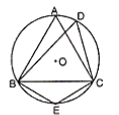Solution:

Given,

ΔABC is an equilateral triangle.

∠ABC = ∠BCA = ∠CAB = 60°

(i) We know that the angles subtended by an arc on the circumference on the same segment are equal.

∠BDC = ∠CAB = 60°

(ii) ABEC is a cyclic quadrilateral.

We know that the sum of opposite angles of a cyclic quadrilateral is supplementary.

∠BAC + ∠BEC = 180°

60° + ∠BEC = 180°

∠BEC = 180° – 60°

∠BEC = 120°

(c) A bucket is raised from a well by means of a rope that is wound around a wheel of diameter 77 cm. Given that the bucket ascends in 1 min 28 sec with a uniform speed of 1. 1 m/sec, calculate the number of complete revolutions the wheel makes in raising the bucket. Take π = 22/7 

Solution:

Given,

Diameter of wheel = 77 cm

Radius of the wheel = r = 77/2 cm = 77/200 m

Time = 1 min 28 sec = (60 + 28) sec = 88 sec

Speed = 1.1 m/s

Distance = Speed × Time

= 1.1 × 88

= 96.8 m

Number of revolutions = Distance/ Circumference of wheel

= 96.8/ 2πr

= 96.8/ [2 × (22/7) × (77/200)]

= 96.8/2.42

= 40

Therefore, the number of complete revolutions the wheel makes in raising the bucket is 40.

Question 10

(a) Prove the following identity:

[1/ (sin θ + cos θ)] + [1/ (sin θ – cos θ)] = 2 sin θ/ (1 – 2 cos2θ) 

Solution:

LHS = [1/ (sin θ + cos θ)] + [1/ (sin θ – cos θ)]

= [(sin θ + cos θ + sin θ – cos θ)/ (sin θ + cos θ)(sin θ – cos θ)]

= 2 sin θ/ (sin2θ – cos2θ)

= 2 sin θ/ [(1 – cos2θ) – cos2θ]

= 2 sin θ/ (1 – cos2θ – cos2θ)

= 2 sin θ/ (1 – 2 cos2θ)

= RHS

(b) The following table shows the distribution of the heights of a group of factory workers:

 Height (in cm) 150 – 155 155 – 160 160 – 165 165 – 170 170 – 175 175 – 180 180 – 185 No. of workers 6 12 18 20 13 8 6

(i) Determine the cumulative frequencies.

(ii) Draw the cumulative frequency curve on a graph paper. 

Solution:

(i) Cumulative frequency table:

 Height (in cm) No. of workers (f) Cumulative frequency (cf) 150 – 155 6 6 155 – 160 12 18 160 – 165 18 36 165 – 170 20 56 170 – 175 13 69 175 – 180 8 77 180 – 185 6 83

(ii) Plot the points (155, 6), (160, 18), (165, 36), (170, 56), (175, 69), (180, 77) and (185, 83) on the graph and then join them with a free hand to obtain a cumulative frequency curve.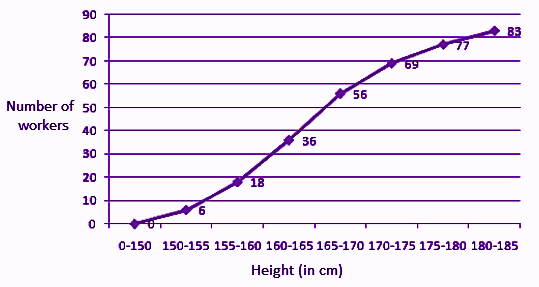Question 11

(a) PAT is a tangent to the circumcircle of ΔABC such that PT || BC. Show that AB = AC. 

Solution:

Given,

PAT is a tangent to the circumcircle of ΔABC such that PT || BC.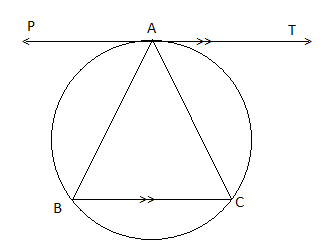∠PAC = ∠ABC….(i) (angles in the alternate segment)

∠PAC = ∠ACB….(ii) (alternate interior angles)

From (i) and (ii),

∠ABC = ∠ACB

We know that the sides opposite to equal angles are equal.

AB = AC

(b) A die is thrown once. What is the probability that the number obtained is:

(i) even?

(ii) other than 4? 

Solution:

Given,

A die is thrown once.

Sample space = S = {1, 2, 3, 4, 5, 6}

n(S) = 6

(i) Let A be the event of getting an even number.

A = {2, 4, 6}

n(A) = 3

P(A) = n(A)/n(S)

= 3/6

= 1/2

(ii) Let B be the event of getting a number other than 4.

B = {1, 2, 3, 5, 6}

n(B) = 5

P(B) = n(B)/ n(S)

= 5/6

(c) Find the equation of the line passing through (-2, -4) and perpendicular to the line 3x – y + 5 = 0. 

Solution:

Given,

3x – y + 5 = 0….(i)

y = 3x + 5

Gradient of the line = 3

The slope of the which is perpendicular to (i) is m = -1/3

The equation of line passing through (-2, -4) and perpendicular to (i) is:

y – (-4) = m[x – (-2)]

y + 4 = (-1/3) (x + 2)

3(y + 4) = -x – 2

3y + 12 + x + 2 = 0

x + 3y + 14 = 0